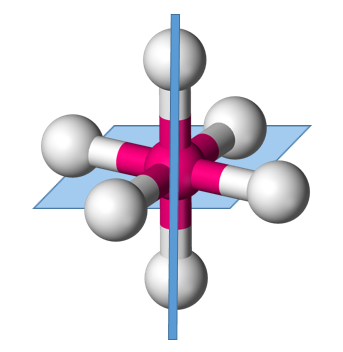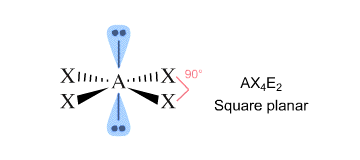# Two different compounds have the formula XeF_2Cl_2. How do you write the Lewis structures for these two compounds, and describe how measurement of dipole moments might be used to distinguish between them?

Dec 4, 2016

Here's how I would do it.

#### Explanation:

Draw the Lewis structure.

Start with a trial structure. Put two $\text{F}$ atoms and two $\text{Cl}$ atoms on a central $\text{Xe}$, and give every atom an octet.The trial structure has 32 valence electrons.

$\text{1 Xe" + "2 F" + "2 Cl} = 8 + 14 + 14 = 36$ valence electrons

We have four extra electrons, so we add 2 lone pairs on the central atom.

The Lewis structure isThis is an ${\text{AX"_4"E}}_{2}$ system.

Its electron geometry is octahedral.The bulky lone pairs will occupy the top and bottom axial positions, and the $\text{F}$ and $\text{Cl}$ atoms will occupy the equatorial positions.The molecular geometry is square planar.

The atoms can arrange themselves in two ways.

In the trans isomer the $\text{F—Xe—F}$ and $\text{Cl—Xe—Cl}$ bond angles are 180 °.The $\text{Xe—F}$ bond dipoles (red) cancel, and the $\text{Xe—Cl}$ bond dipoles cancel.

There is no net dipole, so the trans isomer is nonpolar.

In the cis isomer the $\text{Xe—F}$ and $\text{Xe—Cl}$ bond dipoles do not cancel.The cis isomer has a net dipole moment and the molecule is polar.